`COMPUTE! ISSUE 40 / SEPTEMBER 1983 / PAGE 50`

Caves Of Ice

Marvin Bunker and Robert Tsuk

This award-winning game should provide hours of amusement. Originally written on the Apple, there are also versions here for VIC, 64, Atari, and PET/CBM.

Robert Tsuk invented a game called "QuintiMaze," wrote a version for the Apple, and won a prize in a BYTE magazine game contest - it was published in the September 1982 BYTE. It looked too intriguing to be limited to Apple owners, so I wrote a version called "Caves of Ice" which will run on all Commodore computers (VICs require 8K or more expansion memory). I contacted Robert Tsuk about submitting this version as a joint article to COMPUTE! where other Commodore computer owners could share it. He replied that he was converting it for the Atari. These games are the result of our joint efforts.

The Game Scenario
At the start you are somewhere in a five-story structure made entirely of ice. Each floor has 25 rooms in a five-by-five array. Carved into the walls of each room are one or more openings, doors to the north, south, east, west, up, or down. However, you can see only the doors available to you from your present vantage point. Only one door in the building opens to the outside - it may be in a wall, the roof, or the floor of the building. Your goal is to get out as fast as possible. You could freeze inside these caves of ice.
You can change which direction you are facing at any time - complete instructions are included in the program.
After finding your way out, you are given the option of trying the same maze and same starting point again to see if you can improve your time. Or, you can play again with a new random maze.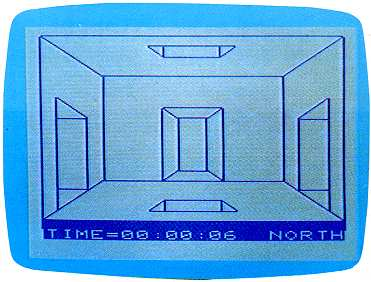A room with five possible exits -only one of the rooms you
may encounter in "Caves of Ice." VIC version.

Strategy
To quote from Robert Tsuk's earlier article: "The strategy for Quinti-Maze is fairly simple; be methodical. Because all the rooms in the maze look similar, you could wander around forever without finding the exit. My favorite method is to travel in one direction as far as I can go, then I assume I'm at one of the outside walls and search there for an exit."

A Variation
Insert this line at the beginning of either Program 1 or 2:
1 X=RND(-π)
This initializes the random number generator with the same seed each time you RUN, so you'll always start with the same maze. You can have the sequence U, W, W, W, N, W, S, W committed to memory and amaze your friends with how rapidly you can find your way out. The figure shows the complete maze produced by this starting seed. Note: This seed produces a different maze on the VIC.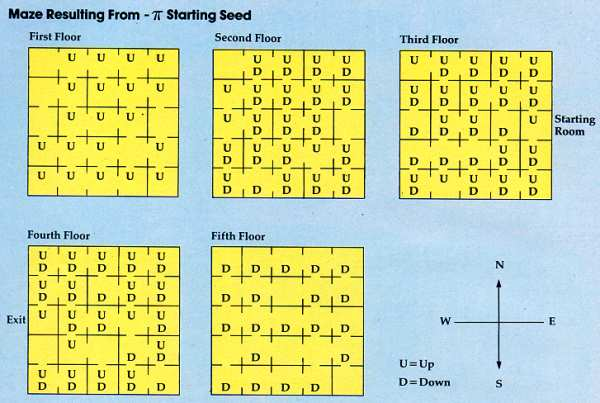Program Notes
In lines 1140 and 3005, the PRINT CHR\$(7) statements produce beeps on the PET when you try to go through a solid wall and when you successfully find your way out. If you have a PET without the built-in beeper, but you do have the CB2 sound, you can replace these statements with the appropriate sequence of POKE statements to give the sound effects desired. VIC and 64 owners should replace the PRINT CHR\$(7) with the proper POKEs to produce sounds on their computers if they desire this feature.
In the INPUT statement in line 2001, following the INSTRUCTIONS are three shifted spaces followed by three left cursors. This is my favorite way to avoid the infamous PET INPUT crash.
Program 1 is Caves of Ice for the VIC (with 8K or more expansion memory) and 64. The only changes required to RUN on PET/CBM models are in the keys which must be typed to produce the graphics in lines 120-151. Program 2 lists these changes for the PET. The graphics are not directly accessible from models with "business style" keyboards. Refer to your manuals for the equivalent CHR\$ codes. Newer CBM models may also require the addition of a line such as:

5 PRINT CHR\$(142)

to put them into graphics mode.

Program 1: Caves of Ice - VIC and 64

10 R\$="{23 RIGHT}"
20 D\$="{23 DOWN}"
25 PRINT"{CLR}"
90 GOSUB2000
100 DIMFC(5,7),FC\$(5)
105 FC\$(1)="NORTH":FC\$(2)="SOUTH":FC\$(3)
=" EAST":FC\$(4)=" WEST"
:NEXT
115 GOTO155
120 PRINT"{CLR} [<20 @>]{DOWN}{LEFT}N
{DOWN}{2 LEFT}N{DOWN}(2 LEFT}N{DOWN}
{2 LEFT}N"
121 PRINT"{HOME}{DOWN}{RIGHT}M{DOWN}M
{DOWN}M{DOWN}M[<12 @>]"
122 PRINT"{HOME}{DOWN}";:FORI=1TO18:PRIN
T"[<M>]{DOWN}{LEFT}";:NEXT:PRINT"
{UP}{RIGHT}N(UP}N{UP}N(UP}N{UP}";
123 PRINT"[<G>]";:FORI=1TO9:PRINT"{UP}
{LEFT}[<G>]";:NEXT
124 PRINT"{HOME}";LEFT\$(D\$,19);"[<M>]
{RVS}{20 SPACES}{OFF}[<G>]{LEFT}{UP}
{LEFT}M{UP}{2 LEFT}M{UP}{2 LEFT}M
{UP}{2 LEFT}M"
125 PRINT"{HOME}"LEFT\$(D\$,15);LEFT\$(R\$,5
)"[<12 T>]"
126 PRINT"{HOME}";LEFT\$(R\$,21);:FORI=ITO
18:PRINT"{DOWN}[<G>]{LEFT}";:NEXT
127 PRINT"{HOME}";LEFT\$(R\$,17);LEFT\$(D\$,
5);:FORI=1TO10:PRINT"[<G>]{DOWN}
{LEFT}";:NEXT
129 RETURN
130 PRINT"{HOME}";LEFT\$(R\$,8);"{DOWN}
[<6 @>]{DOWN}{6 LEFT}M[<G>]
{2 SPACES}[<M>]N{DOWN{5 LEFT}
[<4 T>]":RETURN_
135 PRINT"{HOME}";LEFT\$(D\$,16);LEFT\$(R\$,
9);"<[4 @>]{DOWN}{5 LEFT}N[<G>]
{2 SPACES}[<M>]M{DOWN}{6 LEFT}
[<6 T>]":RETURN
140 PRINT"{HOME}";LEFT\$(D\$,7);"{RIGHT}";
:FORI=1TO11:PRINT"[<M>]{DOWN}{LEFT}"
;:NEXT:PRINT"{RIGHT}{3 UP)[<@>]@"
141 PRINT"{HOME}";LEFT\$(D\$,9);"{2 RIGHT}
[<T>]P";:FORI=1TO5:PRINT"{DOWN}
{LEFT}[<M>]";:NEXT
142 PRINT"{HOME}";LEFT\$(D\$,7);"{2 RIGHT)
M{DOWN}M":RETURN
145 PRINT"{HOME}";LEFT\$(D\$,7);LEFT\$(R\$,9
);"[<4 @>]{DOWN}{5 LEFT}[<M>]M
[<2 @>]N[<G>]";:FORI=1TO5
146 PRINT"{DOWN}{6 LEFT}[<M>] [<G>][<M>]
[<G>]";:NEXT:PRINT"{DOWN}{6 LEFT}
[<M>]N[<2 T>]M[<G>]":RETURN
150 PRINT" {HOME} ";LEFT\$(D\$,7);LEFT\$(R\$,1
9);"N[<G<]{DOWN}{3 LEFT}N [<G>]
{DOWN}{3 LEFT} O[<T>][<G>]";:FORI=1TO5
151 PRINT" {DOWN}{3 LEFT}[<G>] [<G>]";:NE
XT: PRINT" {DOWN}{3 LEFT}L[<@>][<G>]
[DOWN}{LEFT}[<G>]{DOWN}{LEFT}[<G>]":
RETURN
155 DIMS\$(6,6)
165 FORA=1TO5:FORX=1TO5:FORY=1TO5
170 IFA<>5ANDRND(1)<.8THENS\$(X,A)=S\$(X,A
+"O":GOTO180
175 S\$(X,A)=S\$(X,A)+"X"
180 IFMID\$(S\$(X,A-1),(Y-1)*6+1,1)="O"THE
NS\$(X,A)=S\$(X,A)+"O":GOTO190
185 S\$(X,A)=S\$(X,A)+"X"
190 IFY-2<0GOTO200
195 IFMID\$(S\$(X,A),(Y-2)*6+4,1)="O"THENS
\$(X,A)=S\$(X,A)+"O":GOTO205
200 S\$(X,A)=S\$(X,A)+"X"
205 IFY<>5ANDRND(1)<.8THENS\$(X,A)=S\$(X,A
+"O":GOT0215
210 S\$(X,A)=S\$(X,A)+"X"
215 IFX<>5ANDRND(1)<.8THENS\$(X,A)=S\$(X,A
)+"O":GOTO225
220 S\$(X,A)=S\$(X,A)+"X"
225 IFMID\$(S\$(X-1,A),(Y-1)*6+5,1)="O"THE
NS\$(X,A)=S\$(X,A)+"O":GOTO235
230 S\$(X,A)=S\$(X,A)+"X"
235 NEXT:PRINT"*";:NEXT:NEXT
240 X=INT(RND(1)*3)+2:Y=INT(RND(1)*3)+2:
A=INT(RND(1)*3)+2
245 RD=INT(RND(1)*6)+1:ONRDGOTO250,255,2
60,265,270,275
250 A=5:Pl\$=LEFT\$(S\$(X,A),(Y-1)*6):L=29-
LEN(Pl\$):P2\$=RIGHT\$(S\$(X,A),L)
251 S\$(X,A)=P1\$+"O"+P2\$:GOTO290
255 A=1:Pl\$=LEFT\$(S\$(X,A),(Y-1)*6+1):L=2
9-LEN(Pl\$):P2\$=RIGHT\$(S\$(X,A),L)
256 S\$(X,A)=P1\$+"O"+P2\$:GOTO290
260 Y=5:Pl\$=LEFT\$(S\$(X,A),(Y-1)*6+3):L=2
9-LEN(Pl\$):P2\$=RIGHT\$(S\$(X,A),L)
261 S\$(X,A)=P1\$+"O"+P2\$:GOTO290
265 Y=1:Pl\$=LEFT\$(S\$(X,A),(Y-1)*6+2):L=2
9-LEN(Pl\$):P2\$=RIGHT\$(S\$(X,A),L)
266 S\$(X,A)=P1\$+"O"+P2\$:GOTO290
270 X=5:Pl\$=LEFT\$(S\$(X,A),(Y-1)*6+4):L=2
9-LEN(Pl\$):P2\$=RIGHT\$(S\$(X,A),L)
271 S\$(X,A)=P1\$+"O"+P2\$:GOTO290
275 X=1:Pl\$=LEFT\$(S\$(X,A),(Y-1)*6+5):L=2
9-LEN(Pl\$):P2\$=RIGHT\$(S\$(X,A),L)
276 S\$(X,A)=P1\$+"O"+P2\$:GOTO290
290 PRINT:PRINT: PRINT"HIT {RVS}RETURN
{OFF} TO START"
300 GETC\$:IFC\$=""GOTO300
1000 X=INT(RND(1)*5)+l:Y=INT(RND(1)*5)+1
:A=INT(RND(1)*5)+1
1005 SX=X:SY=Y:SA=A
1010 FC=1:TI\$="000000":GOTO1220
1020 PRINT"{HOME}";LEFT\$(D\$,20);LEFT\$(R\$
,16)"{RVS}";FC\$(FC):A\$="":D=0
1030 TX\$=TI\$:TP\$=LEFT\$(TX\$,2)+":"+MID\$(T
X\$,3,2)+":"+RIGHT\$(TX\$,2)
1040 PRINT"{HOME}";LEFT\$(D\$,20);" {RVS}T
IME=";TP\$;"{2 SPACES}"
1050 GETA\$
1060 IFA\$="U"THEND=1
1070 IFA\$="D"THEND=2
1080 IFA\$="N"THEND=3
1090 IFA\$="S"THEND=4
1100 IFA\$="E"THEND=5
1110 IFA\$="W"THEND=6
1120 IFA\$="F"GOTO1280
1130 IFD=0GOTO1020
1140 IFMID\$(S\$(X,A),(Y-1)*6+D,1)<>"O"THE
NPRINTCHR\$(7):GOTO1020
1150 ONDGOTO1160,1170,1180,1190,1200,1210
1160 A=A+1:GOTO1220
1170 A=A-1:GOTO1220
1180 Y=Y-1:GOTO1220
1190 Y=Y+1:GOTO1220
1200 X=X+1:GOTO1220
1210 X=X-1
1220 IFX>50RX<1ORY>50RY<1ORA>50RA<lTHENP
RINT"YOU WIN. PLAY AGAIN?":GOTO3000
1230 GOSUB120
1240 FORII=1T06:IFMID\$(S\$(X,A),(Y-1)*6+I
I,1)="X"THENNEXT:GOTO1020
1250 R=FC(FC,II)+1
1260 ONRGOSUB125,130,135,140,145,150
1270 NEXT:GOTO1020
1280 PRINT"{HOME}";LEFT\$(D\$,22);"NEW FAC
ING, N,S,E,W";
1281 GETC\$:IFC\$=""GOT01280
1282 IFC\$<>"N"ANDC\$<>"S"ANDC\$<>"E"ANDC\$<
>"W"GOT01281
1283 PRINTC\$:IFC\$="N"THENFC=1
1284 IFC\$="S"THENFC=2
1285 IFC\$="E"THENFC=3
1286 IFC\$="W"THENFC=4
1287 GOT01220
2000 PRINTLEFT\$(D\$,8);LEFT\$(R\$,5);"{RVS}
CAVES OF ICE{OFF}"
2001 PRINT"{3 DOWN}DO YOU WANT":INPUT"IN
STRUCTIONS{3 SPACES}{3 LEFT}";Y\$
2002 IFLEFT\$(Y\$,l)<>"Y"THENGOTO2100
2010 PRINT"{CLR}THE OBJECT OF {RVS}CAVES
2011 PRINT OUT OF A 5X5X5 CUBIC":PRINT"M
AZE. IN ONE OF THE
2012 PRINT"ROOMS THERE IS AN EXIT":PRINT
"OUT OF THE MAZE.
2013 PRINT:PRINT"YOU MUST TRY TO FIND IT
":PRINT"IN THE MINIMUM TIME.
2014 PRINT"THE COMMANDS ARE :"
2020 PRINT"{RVS}U{OFF} - UP; {RVS}S{OFF)
- SOUTH;":PRINT"{RVS}D{OFF} - DOWN
; {RVS}E{OFF} - EAST;
2030 PRINT"{RVS}N{OFF} - NORTH; {RVS}W
{OFF) - WEST;"
2040 PRINT"{RVS}F{OFF} TO CHANGE FACING.
2050 PRINT:PRINT"HIT {RVS}RETURN{OFF} TO
GO ON.
2051 GETC\$:IFC\$=""GOTO2051
2060 PRINT"{CLR}{RVS}F{OFF} WILL COME BA
CK WITH A":PRINT"QUESTION AS TO WHI
CH
2062 PRINT"FACING YOU WISH. HIT":PRINT"O
NLY ONE KEY":PRINT"AND {RVS}RETURN
{OFF}"
RINT"SECONDS WHILE I SET UP":PRINT"
THE MAZE.
2101 RETURN
3000 PRINT"{3 SPACES}{RVS}Y{OFF} OR
{RVS}N{OFF}?"
3005 FORI=1TO10:PRINTCHR\$(7);:NEXT
3010 GETC\$:IFC\$=""GOTO3010
3020 IFC\$<>"Y"ANDC\$<>"N"GOTO3010
3030 IFC\$="N"THENSTOP
3032 PRINT"SAME MAZE {RVS}S{OFF} OR
3033 PRINT"NEW MAZE {RVS}N{OFF}?"
3034 GETC\$:IFC\$=""GOTO3034
3035 IFC\$<>"S"ANDC\$<>"N"GOTO3034
3036 IFC\$="N"GOTO165
3040 X=SX:Y=SY:A=SA:GOTO1010
4000 DATA1,2,4,0,5,3,1,2,0,4,3,5,1,2,3,5
,4,0,1,2,5,3,0,4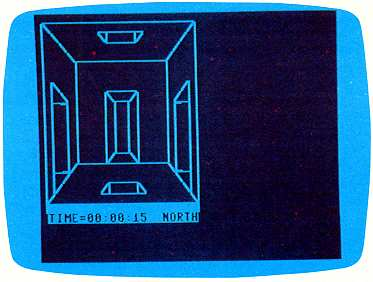64 version.

Program 2:
Caves Of Ice - Changes For PET/CBM

120 PRINT"{CLR} \$\$\$\$\$\$\$\$\$\$\$\$\$\$\$\$\$\$\$
{DOWN}{LEFT}N{DOWN}{2 LEFT}N{DOWN}
{2 LEFT}N{DOWN}{2 LEFT}N"
121 PRINT"{HOME}{DOWN}{RIGHT}M{DOWN}M
{DOWN}M{DOWN}M\$\$\$\$\$\$\$\$\$\$\$\$"
122 PRINT"{HOME}{DOWN}";:FORI=1TO18:PRIN
T"'{DOWN}{LEFT}";:NEXT:PRINT"{UP}
{RIGHT}N{UP}N{UP)N{UP)N{UP}";
123 PRINT"%";:FORI=1T09:PRINT"{UP}{LEFT}
%";:NEXT
124 PRINT"{HOME}";LEFT\$(D\$,19);"'{RVS}
{20 SPACES}{OFF}%{LEFT}{UP}{LEFT}M
{UP}{2 LEFT}M{UPT{2 LEFT}M{UP}
{2 LEFT}M"
125 PRINT"{HOME}"LEFT\$(D\$,15);LEFT\$(R\$,5
);"###########"
126 PRINT"{HOME}";LEFT\$(R\$,21);:FORI=ITO
18:PRINT"{DOWN}%{LEFT}";:NEXT
127 PRINT"{HOME}";LEFT\$(R\$,17);LEFT\$(D\$,
5);:FORI=1TO10:PRINT"%{DOWN}{LEFT}";
:NEXT
129 RETURN
130 PRINT"{HOME}";LEFT\$(R\$,8);"{DOWN}\$\$\$
\$\$\${DOWN}{6 LEFT}M%{2 SPACES}'N
{DOWN]{5 LEFT}####":RETURN
135 PRINT"{HOME}";LEFT\$(D\$,16);LEFT\$(R\$,
9);"\$\$\$\${DOWN}{5 LEFT}N%{2 SPACES}'M
{DOWN}{6 LEFT}######":RETURN
140 PRINT"{HOME}";LEFT\$(D\$,7);"{RIGHT}";
:FORI=1TO11:PRINT"'{DOWN}{LEFT}";:NE
XT:PRINT"{RIGHT}{3 UP}\$:"
141 PRINT"{HOME}";LEFT\$(D\$,9);"{2 RIGHT}
#P";:FORI=1TO5:PRINT"{DOWN}{LEFT}'";
:NEXT
142 PRINT"{HOME}";LEFT\$(D\$,7);"{2 RIGHT)
M{DOWN}M":RETURN
145 PRINT"{HOME}";LEFT\$(D\$,7);LEFT\$(R\$,9
);"\$\$\$\${DOWN}{5 LEFT}'M\$\$N%";:FORI=1
TO5
146 PRINT"{DOWN}{6 LEFT}' %' %";:NEXT:PR
INT"{DOWN}{6 LEFT}'N##M%":RETURN
150 PRINT"{HOME}";LEFT\$(D\$,7);LEFT\$(R\$,1
9);"N%{DOWN}{3 LEFT}N %{DOWN}
{3 LEFT}O#%";:FORI=1TO5
151 PRINT"{DOWN}{3 LEFT}% %";:NEXT:PRINT
"{DOWN}{3 LEFT}L\$%{DOWN}{LEFT}%
{DOWN}{LEFT}%":RETURN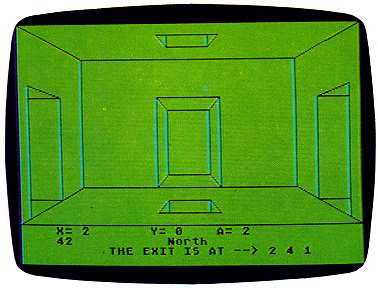"Caves of Ice" Atari version.

Program 3: Caves Of Ice -Atari Version

10 GOTO 100
15 L=PEEK(708):POKE 708,PEEK(710):PO
KE 710,2
16 B=B+AB:IF B=1 OR B=5 THEN AB=AB*-
1
17 T=T+INT(RND(0)*3-1):IF T<1 THEN T
=5
18 IF T>5 THEN T=1
20 SPOT=6*X+30*Y+150*A+I:RETURN
30 COLOR 1
32 ? #6;"(CLEAR}":PLOT 0,0:DRAWTO 59
,29:DRAWTO 59,129:DRAWTO 259,129:
DRAWTO 259,29:DRAWTO 59,29:PLOT 3
19,0:DRAWTO 259,29
35 PLOT 319,159:DRAWTO 259,129:PLOT
0,159:DRAWTO 59,129:PLOT 0,159:DR
AWTO 319,159:SETCOLOR 2,A+11,10:R
ETURN
40 PLOT 129,9:DRAWTO 189,9:DRAWTO 17
9,19:DRAWTO 139,19:DRAWTO 129,9:P
LOT 139,9:DRAWTO 139,19:PLOT 179,
9:DRAWTO 179,19
45 RETURN
50 PLOT 129,149:DRAWTO 189,149:DRAWT
O 179,139:DRAWTO 139,139:DRAWTO 1
29,149:PLOT 139,139:DRAWTO 139,14
9
55 PLOT 179,139:DRAWTO 179,149:RETUR
N
60 PLOT 279,59:DRAWTO 309,49:DRAWTO
309,154:DRAWTO 279,139:DRAWTO 279
,59:DRAWTO 309,59:PLOT 279,139
65 DRAWTO 309,139:RETURN
70 PLOT 9,49:DRAWTO 39,59:DRAWTO 39,
139:DRAWTO 9,154:DRAWTO 9,49:PLOT
9,59:DRAWTO 39,59:PLOT 9,139
75 DRAWTO 39,139:RETURN
80 PLOT 129,59:DRAWTO 189,59:DRAWTO
189,129:DRAWTO 129,129:DRAWTO 129
,59:DRAWTO 139,69:DRAWTO 179,69
82 DRAWTO 179,119:DRAWTO 139,119:DRA
WTO 139,69:PLOT 179,69:DRAWTO 189
,59:PLOT 189,129:DRAWTO 179,119:P
LOT 129,129
85 DRAWTO 139,119:RETURN
90 RETURN
100 DIM A\$(750),G\$(1),FC(4,6),SC\$(20
0):A\$(750)="O":FOR I=20 TO 192 S
TEP 11:SC\$(I)="->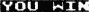<-":NEXT
I
101 SC\$(1,19)="{19 SPACES}":SC\$(LEN(S
C\$)+1)="{10 SPACES}"
102 GOSUB 3000
105 FOR FC=1 TO 4:FOR I=1 TO 6:READ
A:FC(FC,I)=A:NEXT I:NEXT FC
107 X=0
109 Y=0
110 A=0
115 GOSUB 250
120 IF RND(0)<0.7 AND A<4 THEN I=1:G
OSUB 20:A\$(SPOT,SPOT)="X"
130 IF RND(0)<0.7 AND X<4 THEN I=3:G
OSUB 20:A\$(SPOT,SPOT)="X"
140 IF RND(0)<0.7 AND Y<4 THEN I=5:G
OSUB 20:A\$(SPOT,SPOT)="X"
150 IF A>0 THEN A=A-1:I=1:GOSUB 20:A
=A+I:IF A\$(SPOT,SPOT)="X" THEN I
=2:GOSUB 20:A\$(SPOT,SPOT)="X"
160 IF X>0 THEN X=X-1:I=3:GOSUB 20:X
=X+1:IF A\$(SPOT,SPOT)="X" THEN I
=4:GOSUB 20:A\$(SPOT,SPOT)="X"
170 IF Y>0 THEN Y=Y-1:I=5:GOSUB 20:Y
=Y+1:IF A\$(SPOT,SPOT)="X" THEN I
=6:GOSUB 20:A\$(SPOT,SPOT)="X"
190 A=A+1:IF A<5 THEN 115
191 Y=Y+1:IF Y<5 THEN 110
192 X=X+1:IF X<5 THEN 109
193 SOUND 0,0,0,0:SOUND 1,0,0,0
195 GOTO 300
200 GOSUB 30:FOR Q=1 TO 6:1=Q
210 GOSUB 20:IF A\$(SPOT,SPOT)<>"X" T
HEN 230
215 I=FC(F,Q)
220 ON I GOSUB 40,50,60,70,80,90
230 NEXT Q:RETURN
250 L=PEEK(708):POKE 708,PEEK(709):P
OKE 709,PEEK(710):POKE 710,2
255 SOUND 0,BASS(O,B),10,10:SOUND 1,
TREBLE(O,T),10,14
260 B=B+AB:IF B=1 THEN 0=0+1:AB=1:IF
O=4 THEN O=1
270 IF B=5 THEN AB=AB*-1
280 T=T+INT(RND(0)*3-1):IF T>5 THEN
T=1
290 IF T<1 THEN T=5
295 RETURN
300 OPEN #1,4,0,"K:"
305 GRAPHICS 8
310 X=INT(RND(0)*5)
315 Y=INT(RND(0)*5)
320 A=INT(RND(0)*5)
330 I=INT(RND(0)*6+1):ON I GOTO 335,
340,3345,350,355,360
335 A=4:GOTO 370
340 A=0:GOTO 370
345 X=4:GOTO 370
350 X=0:GOTO 370
355 Y=4:GOTO 370
360 Y=0
370 GOSUB 20:A\$(SPOT,SPOT)="X":SX=X:
SY=Y:SA=A
375 X=INT(RND(0)*5)
380 Y=INT(RND(0)*5)
385 A=INT(RND(0)*5)
390 POKE 19,0:POKE 20,0
400 POKE 752,1:SETCOLOR 1,0,0
410 F=1:60SUB 200
420 SETCOLOR 1,0,0
500 IF PEEK(764)=255 THEN 1000
510 GET #1,G:G\$=CHR\$(G)
515 D=0
520 IF G\$="U" THEN D=1
525 IF G\$="*" THEN TELLIT=1
530 IF G\$="D" THEN D=2
535 IF G\$="?" THEN SHOWIT=1
540 IF G\$="E" THEN D=3
545 TRAP 545:IF G\$="F" THEN ? "
{CLEAR}(DOWN)":INPUT FB:IF FB<5
AND FB>O THEN F=FB:GOSUB 200:GOT
O 1000
550 IF G\$="W" THEN D=4
560 IF G\$="N" THEN D=5
570 IF G\$="S" THEN D=6
575 IF D<1 OR D>7 THEN 500
580 I=D:GOSUB 20
590 IF A\$(SPOT,SPOT)<>"X" THEN GOSUB
900:GOTO 1000
600 ON D GOTO 605,610,615,620,625,63
0
605 A=A+I:GOTO 640
610 A=A-1:GOTO 640
615 X=X+1:GOT0 640
620 X=X-1:GOTO 640
625 Y=Y+1:GOTO 640
630 Y=Y-1
640 IF A<0 OR A>4 OR X<0 OR X>4 OR Y
<0 OR Y>4 THEN 2000
650 GOSUB 200
660 GOTO 1000
900 FOR Q=1 TO 4:CC=(CC=O)*14:POKE 7
10,CC:SOUND 0,CC*7+60,CC,10:FOR
W=1 TO 10:NEXT W:NEXT Q
910 SOUND 0,0,0,0:SETCOLOR 2,A+11,10
:SETCOLOR 1,0,0:RETURN
1000 TRAP 40000:GOSUB 1400:GOSUB 130
0:IF TIME2=TIME THEN GOTO 500
1005 POKE 657,4:POKE 656,1:? "
C3 SPACES)(3 LEFT1";TIME:TIME2=
TIME:IF TELLIT=0 THEN 500
1010 POKE 656,0:POKE 657,4:? "X= ";X
;"{TAB)-Y= ";Y;"(TAB)A= ";A;:IF
SHOWIT=0 THEN 500
1020 SHOWIT=0:POKE 656,2:POKE 657,10
:? "THE EXIT IS AT --> ";SX;" "
;SY;" ";SA;:GOTO 500
1300 TIME=INT(4.25*PEEK(19)+PEEK(20)
/60):RETURN
1400 POKE 657,17:POKE 656,1:ON F GOS
UB 1405,1410,1415,1420:RETURN
1405 ? "North":RETURN
1410 ? "South":RETURN
1415 ? "East":RETURN
1420 ? "West":RETURN
2000 GRAPHICS 2+16:SETCOLOR 1,4,12:S
ETCOLOR 2,15,8:SETCOLOR 3,10,4:
SETCOLOR 0,0,15
2005 C=1
2010 FOR I=1 TO 1S
2020 C=C+1:IF C>3 THEN C=1
2030 ON C GOTO 2032,2035,2037
2032 COLOR 10:GOTO 2040
2035 COLOR 170:GOTO 2040
2037 COLOR 138:GOTO 2040
2040 PLOT I,O:PLOT I,11
2043 TRAP 2050
2045 PLOT 0,I:PLOT 19,1
2050 NEXT I
2055 POSITION 0,0:? #6;"(J)":POSITIO
N 19,0:? #6;"(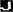}"
2060 POSITION 5,2:? #6;"YOU ESCAPED"
2065 POSITION 4,3:? #6;"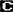VES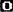F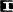CE"
2070 POSITION 4,7:? #6;"IN ";TIME;"
SECONDS"
2080 POSITION 5,9:? #6;"HIT ANY KEY"
2090 I=1
2100 L=PEEK(709):POKE 709,PEEK(710):
POKE 710,PEEK(711):POKE 711,L
2105 GOSUB 255:POSITION 1,5:? #6;SC\$
(I,I+17):I=I+1:IF I>180 THEN I=
1
2107 IF PEEK(764)<>255 THEN 2200
2110 FOR W=1 TO 15:NEXT W:GOTO 2100
2200 POKE 764,255:GRAPHICS 0:POSITIO
N 4,4:SOUND 0,0,0,0:SOUND 1,0,0
,0
2210 ? "PLAY AGAIN ";:INPUT A\$
2220 IF A\$(1,1)="Y" THEN RUN
2230 END
3000 GRAPHICS 18
3010 POSITION 7,4:PRINT #6;"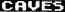"
3020 POSITION 6,7:PRINT #6;"of ice"
3030 COLOR 138
3040 PLOT 1,1:DRAWTO 18,1
3050 DRAWTO 18,10:DRAWTO 1,10:DRAWTO
1,1
3060 AB=1
3070 DIM BASS(3,5),TREBLE(3,5)
3080 RESTORE 3100
3090 FOR I=1 TO 3:FOR T=1 TO 5:READ
B,TR:BASS(I,T)=B:TREBLE(I,T)=TR
:NEXT T:NEXT I:T=1:B=1:0=1:RETU
RN
3100 DATA 243,121,19-3,96,162,81,144,
72,136,68
3110 DATA 182,91,144,72,121,60,108,5
3,102,50
3120 DATA 162,81,128,64,108,53,96,47
,91,45
3130 DATA 1,2,3,4,5,6,1,2,4,3,6,5,1,
2,5,6,4,3, 1,2,6,5,3,4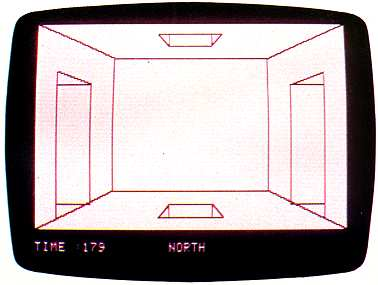A room with four possible exits. Apple version of "Caves
of Ice."

Program 4: Caves Of Ice-Apple Version

1 DATA 201,84,208,15,32,177,0,32,248,
230,138,72,32,183,0,201,44,240,3,7
6,201,222,32,177,0,32,248,230
2 FOR I = 768 TO 833: READ P: POKE I,P
: NEXT I
3 DATA 104,134,3,134,1,133,0,170,160,
1,132,2,173,48,192,136,208,4,198
4 DATA 1,240,7,202,208,246,166,0,208,
239,165,3,133,1,198,2,208,241,96
5 POKE 1013,76: POKE 1014,0: POKE 1015
,3
10 TEXT : HOME
90 GOSUB 2000
100 DIM FC(5,7): DIM FC\$(5)
105 FC\$(1) = "NORTH": FC\$ (2) = "SOUTH":F
C\$(3) = "EAST":FC\$(4) = "WEST"
110 FOR B = 1 TO 4: FOR I = 1 TO 6: READ
FC(B,I): NEXT : NEXT
115 SOTO 155
120 HPLOT 0,0 TO 279,0 TO 279,159 TO 0
,159 TO 0,0 TO 69,29 TO 209,29 TO
209,129 TO 69,129 TO 69,29: HPLOT
209,29 TO 279,0: HPLOT 209,129 TO
279,159: HPLOT 69,129 TO 0,159: RETURN
125 RETURN
130 HPLOT 109,9 TO 169,9 TO 159,19 TO
119,19 TO 109,9: HPLOT 119,19 TO 1
19,9: HPLOT 159,19 TO 159,9: RETURN
135 HPLOT 119,139 TO 159,139 TO 169,14
9 TO 109,149 TO 119,139: HPLOT 119
,139 TO 119,149: HPLOT 159,139 TO
159,149: RETURN
140 HPLOT 19,39 TO 49,49 TO 49,139: HPLOT
19,149 TO 19,39: HPLOT 19,139 TO 4
9,139: HPLOT 19,49 TO 49,49: RETURN
145 HPLOT 119,59 TO 159,59 TO 159,129 TO
119,129 TO 119,59 TO 129,69 TO 149
,69 TO 149,119 TO 129,119 TO 129,6
9: HPLOT 149,69 TO 159,59: HPLOT 1
49,119 TO 159,129: HPLOT 129,119 TO
119,129: RETURN
150 HPLOT 229,49 TO 259,39 TO 259,149:
HPLOT 229,139 TO 229,49: HPLOT 22
9,49 TO 259,49: HPLOT 229,139 TO 2
59,139: RETURN
155 DIM S\$(6,6)
160 INPUT "RESTART OLD MAZE ";Y\$: IF LEFT\$
(Y\$,1) _ "Y" THEN 1360
165 FOR A = 1 TO 5: FOR X = 1 TO 5: FOR
Y = 1 TO 5
167 & T10 * A + 10 * X + 10 * Y,10
170 IF A < > 5 AND RND (1) < .80 THEN
S\$(X,A) = S\$(X,A) + "0": GOTO 180
175 S\$(X,A) = S\$(X,A) + "X"
180 IF MID\$ (S\$(X,A - 1),(Y - 1) * 6 +
1,1) = "0" THEN S\$(X,A) = S\$(X,A) +
"0": GOTO 190
185 S\$(X,A) = S\$(X,A) + "X"
190 IF Y - 2 < 0 THEN 200
195 IF MID\$ (S\$(X,A),(Y - 2) * 6 + 4,
1) = "0" THEN S\$(X,A) = S\$(X,A) +
"0": GOTO 205
200 S\$(X,A) = S\$(X,A) + "X"
205 IF Y < > 5 AND RND (1) < .8 THEN
S\$(X,A) = S\$(X,A) + "0": GOTO 215
210 S\$(X,A) = S\$(X,A) + "X"
215 IF X < > 5 AND RND (1) < .8 THEN
S\$(X,A) = S\$(X,A) + "0": GOTO 225
220 S\$(X,A) = S\$(X,A) + "X"
225 IF MID\$ (S\$(X - 1,A),(Y - 1) * 6 +
5,1) = "0" THEN S\$(X,A) = S\$(X,A) +
"0": GOTO 235
230 S\$(X,A) = S\$(X,A) + "X"
235 NEXT : NEXT : NEXT
240 X = INT ( RND (1) * 3) + 2:Y = INT
( RND (1) * 3) + 2:A = INT ( RND
(1) * 3) + 2
245 RD = INT ( RND (1) * 6) + 1: ON RD
GOTO 250,255,260,265,270,275
250 A = 5:P1\$ = LEFT\$ (S\$(X,A),(Y - 1)
* 6):L = 29 - LEN (P1\$):P2\$ = RIGHT\$
(S\$(X,A),L):S\$(X,A) = P1\$ + 110" +
P2\$: GOTO 280
255 A = 1:P1\$ = LEFT\$ (S\$(X,A),(Y - 1)
* 6 + 1):L = 29 - LEN (P1\$):P2\$ _
RIGHT\$ (S\$(X,A),L):S\$(X,A) = P1\$ +
"0" + P2\$: GOTO 280
260 Y = 5:P1\$ = LEFT\$ (S\$(X,A),(Y - 1)
* 6 + 3):L = 29 - LEN (P1\$):P2\$ _
RIGHT\$ (S\$(X,A),L):S\$(X,A) = P1\$ +
"0" + P2\$: GOTO 280
265 Y = 1:P1\$ = LEFT\$ (S\$(X,A),(Y - 1)
* 6 + 2):L = 29 - LEN (P1\$):P2\$ _
RIGHT\$ (S\$(X,A),L):S\$(X,A) = P1\$ +
"O" + P2\$: GOTO 280
270 X = 5:P1\$ = LEFT\$ (S\$(X,A),(Y - 1)
# 6 + 4):L = 29 - LEN (P1\$):P2\$ _
RIGHT\$ (S\$(X,A),L):S\$(X,A) = Pi\$ +
"O" + P2\$: GOTO 280
275 X = i:Pl\$ = LEFT\$ (S\$(X,A),(Y - 1)
# 6 + 5):L = 29 - LEN (P1\$):P2\$ _
RIGHT\$ (S\$(X,A),L)tS\$(X,A) - P1\$
"O" + P2\$: GOTO 280
280 SX    =    X: SY = Y: SA ~ A
290 VTAB 23: PRINT "HIT ANY KEY TO STA
RT"
300 IF PEEK ( - 16384) < 127 THEN 300
310 POKE - 16368,00
1000 X = INT ( RND (1) * 5) + 1:Y = INT
( RND (1) # 5) + 1:A = INT ( RND
(1) * 5) + 1:FC = 1: GOTO 1220
1010 HOME : VTAB 22: HTAB 18: PRINT FC
\$(FC):A\$ = "":D = 0: IF LS = 1 THEN
PRINT X,Y,A
1020 VTAB 22: PRINT "TIME :";T: FOR TI
ME = 1 TO 80
1025 IF PEEK ( - 16384) > 127 THEN 10
30
1027 NEXT :T = T + 1: VTAB 22: PRINT "
TIME :";T: GOTO 1020
1030 GET A\$
1035 IF A\$ = "*" THEN LS = 1
1040 IF A\$ = "Q" THEN 1300
1050 IF A\$ = "U" THEN D = 1
1060 IF A\$ = "D" THEN D = 2
1070 IF A\$ = "N" THEN D = 3
1080 IF A\$ = "S" THEN D = 4
1090 IF A\$ = "E" THEN D = 5
1100 IF A\$ = "?" THEN 1290
1110 IF A\$ = "W" THEN D = 6
1120 IF A\$ = "F" THEN GOTO 1280
1130 IF D = 0 THEN 1010
1135 T = T + 1
1140 IF MID\$ (S\$(X,A),(Y - 1) * 6 + D
,1) < > "O" THEN PRINT CHR\$ (7)
: GOTO 1010
1150 ON D GOTO 1160,1170,1180,1190,120
0,1210
1160 A = A + 1: GOTO 1220
1170 A = A - 1: GOTO 1220
1180 Y = Y - 1: GOTO 1220
1190 Y = Y + 1: GOTO 1220
1200 X = X + 1: GOTO 1220
1210 X = X - 1: GOTO 1220
1220 IF X > 5 OR X < 1 OR Y > 5 OR Y <
1 OR A > 5 OR A < 1 THEN PRINT "Y
OU WIN": & T100,100: & T100,50: &
T100,50: & T75,66: & T100,66: & T7
5,66: & T60,255: GOTO 3000
1230 HGR : HCOLOR= 3: HPLOT 0,0: CALL
62454: HCOLOR= 0: GOSUB 120
1240 FOR I = 1 TO 6: IF MID\$ (S\$(X,A)
,(Y - 1) *6+ I,1) = "X" THEN NEXT
: GOTO 1010
1250 R = FC(FC,I) + 1
1260 HCOLOR= 0: ON R GOSUB 125,130,135
,140,145,150
1270 NEXT : GOTO 1010
1280 INPUT "WHAT FACING 1-N 2-S 3-E 4-
W";FC: IF FC < 1 OR FC > 4 THEN 1280
1285 GOTO 1220
1290 INVERSE : HTAB 18: PRINT SX;" ";
SY;" ";SA: NORMAL : GOTO 1220
1300 PRINT "DO YOU WANT TO SAVE THIS M
AZE": INPUT Y\$: IF LEFT\$ (Y\$,1) <
> "Y" THEN GOTO 3000
1310 INPUT "WHAT DO YOU WANT TO CALL I
T ";N\$
1320 D\$ = CHR\$ (4)
1330 PRINT D\$;"OPEN OLD MAZE/";N\$: PRINT
D\$;"WRITE OLD MAZE/";N\$
1340 FOR A1 = 1 TO 5: FOR X1 = 1 TO 5:
PRINT S\$(X1,A1): NEXT : NEXT : PRINT
X: PRINT Y: PRINT A: PRINT T: PRINT FC
1350 PRINT D\$;"CLOSE OLD MAZE/";N\$: GOTO
3000
1360 INPUT "WHAT IS ITS NAME ";N\$
1370 D\$ = CHR\$ (4)
1380 PRINT D\$;"OPEN OLD MAZE/";N\$: PRINT
1390 FOR A1 = 1 TO 5: FOR X1 = 1 TO 5:
INPUT S\$(XI,Al): NEXT : NEXT : INPUT
X: INPUT Y: INPUT A: INPUT T: INPUT FC
1400 PRINT D\$;"CLOSE OLD MAZE/";N\$: GOTO
1220
2000 VTAB 12: HTAB 18: INVERSE : PRINT
"MAZE": NORMAL : VTAB 22: INPUT "D
O YOU WANT INSTRUCTIONS ";Y\$: IF LEFT\$
(Y\$,1) < > "Y" THEN RETURN
2010 HOME : PRINT "THE OBJECT OF MAZE
IS TO FIND YOUR WAY": PRINT : PRINT
"OUT OF A 5X5X5 CUBIC MAZE. IN ONE
OF THE": PRINT "ROOMS THERE IS AN
EXIT OUT OF THE MAZE."
2020 PRINT : PRINT "YOU MUST TRY TO FI
ND IT IN AS FEW TURNS ": PRINT "AS
POSSIBLE. THE COMMANDS ARE ."
2030 PRINT : HTAB 6: INVERSE : PRINT "
U";: NORMAL : PRINT "-UP";: HTAB 1
7: INVERSE : PRINT "S";: NORMAL : PRINT
"-SOUTH"
2040 PRINT : HTAB 6: INVERSE : PRINT "
D";: NORMAL : PRINT "-DOWN";: HTAB
17: INVERSE : PRINT "E";: NORMAL
PRINT "-EAST"
2050 PRINT : HTAB 6: INVERSE : PRINT "
N";: NORMAL : PRINT "-NORTH";: HTAB
17: INVERSE : PRINT "W";: NORMAL
PRINT "-WEST"
2060 PRINT : HTAB 6: INVERSE : PRINT "
Q";: NORMAL : PRINT "-QUIT";: HTAB
17: INVERSE : PRINT "F";: NORMAL
PRINT "-CHANGE FACING"
2070 VTAB 23: PRINT "HIT ";: INVERSE
PRINT "SPACE";: NORMAL : PRINT "
FOR MORE"
2080 IF PEEK ( - 16384) < 127 THEN 2080
2090 POKE - 16368,0: HOME : INVERSE
PRINT "F";: NORMAL : PRINT " WILL
COME BACK WITH A QUESTION AS TO":
PRINT : PRINT "WHICH FACING YOU W
ISH.HIT ONLY ONE KEY": PRINT : PRINT
"AND ";: INVERSE : PRINT "RETURN":
NORMAL
2100 PRINT : PRINT "PLEASE WAIT WHILE
IT SETS UP THE MAZE": PRINT : PRINT
: RETURN
3000 TEXT : HOME : VTAB 5: HTAB 12: PRINT
"CONGRATULATIONS !"
3010 PRINT : PRINT TAB( 7)"YOU HAVE F
INISHED THE MAZE IN ": PRINT TAB(
7) T;" SECONDS"
3030 INPUT "DO YOU WANT TO PLAY AGAIN
? ";Y\$
3040 IF LEFT\$ (Y\$,1) = "Y" THEN RUN
9999 NORMAL
10000 DATA 1,2,4,0,5,3,1,2,0,4,3,5
,1,2,3,5,4,0,1,2,5,3,0,4

 Atari And Apple Versions Robert Tsuk When I received Marv Bunker's letter, I agreed a version should be made available for Commodore owners. Also, as an Atari owner, I wanted to include a version for that computer, too. The lack of dimensioned string variables on the Atari made it tricky, but, as evidenced by Program 3, it was successfully adopted.     If you find the game too challenging, the Atari and Apple versions have several features not found in the Commodore version which may be of assistance. If you type an  * the program will tell you your location in the maze. You are given your X and Y coordinates (0-4) on the current level, and a value for A, which indicates which level (0-4) you are currently on. If you get really lost, you can find your location by typing the *, then a ?. The program will briefly display the X, Y, and A coordinates of the exit. But remember, using the ? is frowned upon unless you're really lost.     As in the Commodore version, you move through the maze by typing the N, S, E, W, U, and D keys to specify the direction of movement. However, if you type F to change the direction you are facing, you must then type a number instead of a letter to specify the new direction you wish to face. You'll need to remember that N =1, S = 2, E = 3, and W=4.     The Apple version of Caves of Ice (Program 4), the original Quinti-Maze, uses almost 48K. It includes a SAVE the game feature. Special attention must be paid to the first five lines and the data therein, as a mistake in the data will cause a crash in line 167.     The Atari version uses just over 16K. Although it has no SAVE the game feature, it has some pretty flashy graphics and sound.